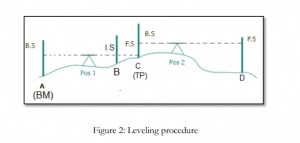# DIFFERENTIAL OR FLY LEVELLING – REDUCE LEVELS BY H.I METHOD

## DIFFERENTIAL OR FLY LEVELLING –REDUCE LEVELS BY H.I METHOD

In this post, we are going to discuss about to find the difference in elevation and calculate the reduced levels of various points by H.I method.

## Types of equipment used to find differential levelling

Dumpy level, Tripod, Leveling staff, staff bubble.

## Procedure for differential levelling using reduce levels by H.I Method

Suppose that B, C and D are points whose reduced level is to be determined as in Figure 1:1. Place the staff over a benchmark (BM), whose reduced level is known, and set up the
instrument in a convenient and safe location where the BM (point A) is visible. Take a sight
on the staff, that reading is called backsight (B.S). See Figure 2
2. Place staff over B. Take a sight on the staff, that reading is called Intermediate sight (I.S). See
figure 2
3. Place staff over C. Since the distance between the level and D is long, so it is not possible to
read the staff over D from the current level position (pos 1). So take the last reading over C
for this position, this will be a foresight (F.S). See figure 2
4. Now, move the instrument to a new position (position 2) and take the reading on the
previous position of the staff (over C). This position of staff is known as Turning Point
(T.P) and this reading will be backsight (BS). See figure 2
5. Now, shift the staff to point D and take the reading, this reading will be foresight (F.S). See
figure 2Tabulation### Calculation for differential levelling using H.I Method

1. The height of instrument position(1) can be calculated as:
HI of position 1 = Elevation of BM + BS at BM
2. The reduced level of any point (i) taken from position 1, can be calculated as:
R.L of point i = HI of position 1 – staff reading at point i
3. The height of instrument position(2) can be calculated as:
HI of position 2 = Reduced level of TP1 + BS at TP1
4. The reduced level of any point (i) taken from position 2, can be calculated as:
R.L of point i = HI of position 2 – staff reading at point i
5. And so on….

6. Checks:

The following checks on the booking and arithmetic calculations are performed:
A) Number of BS readings = Number of FS readings
B) ∑BS – ∑FS = RL of last point – RL of first point

Hope you like this post, differential levelling using reduce levels by H.I Method.

Open